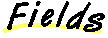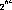`Packages  This Package  Prev  Next  Index  `

# §1.9 Class Long

```public  final  class  java.lang.Long
extends  java.lang.Number  (I-§1.11)
{
// Fields
public final static long MAX_VALUE;	§1.9.1
public final static long MIN_VALUE;	§1.9.2

// Constructors
public Long(long  value);	§1.9.3
public Long(String  s);	§1.9.4

// Methods
public double doubleValue();	§1.9.5
public boolean equals(Object  obj);	§1.9.6
public float floatValue();	§1.9.7
public static Long getLong(String  nm);	§1.9.8
public static Long getLong(String  nm, long  val);	§1.9.9
public static Long getLong(String  nm, Long  val);	§1.9.10
public int hashCode();	§1.9.11
public int intValue();	§1.9.12
public long longValue();	§1.9.13
public static long parseLong(String  s);	§1.9.14
public static long parseLong(String  s, int  radix);	§1.9.15
public static String toBinaryString(long  i);	§1.9.16
public static String toHexString(long  i);	§1.9.17
public static String toOctalString(long  i);	§1.9.18
public String toString();	§1.9.19
public static String toString(long  i);	§1.9.20
public static String toString(long  i, int  radix);	§1.9.21
public static Long valueOf(String  s);	§1.9.22
public static Long valueOf(String  s, int  radix);	§1.9.23
}
```
This class wraps a value of the primitive type long in an object. An object of type Long contains a single field whose type is long.

In addition, this class provides a number of methods for converting a long to a String and a String to a long, as well as other constants and methods useful when dealing with a long.

##### MAX_VALUE

```public final static long MAX_VALUE = 9223372036854775807L ```
The largest value of type long.

### MIN_VALUE

```public final static long MIN_VALUE = -9223372036854775808L ```
The smallest value of type long.

##### Long

```public Long(long value) ```
Constructs a newly allocated Long object that represents the primitive long argument.
Parameters:
```value - ```the value to be reprsented by the Long

### Long

```public Long(String s) ``` ```throws NumberFormatException ```
Constructs a newly allocated Long object that represents the value represented by the string. The string is converted to an long value as if by the valueOf method (I-§1.9.22).

Parameters:
```s - ```the string to be converted to a Long
Throws
NumberFormatException (I-§1.41)
If the String does not contain a parsable long integer.

##### doubleValue

```public double doubleValue() ```
Returns:
The long value represented by this object is converted to type double and the result of the conversion is returned.
Overrides:
doubleValue in class Number (I-§1.11.1).

### equals

```public boolean equals(Object obj) ```
The result is true if and only if the argument is not null and is a Long object that contains the same long value as this object.
Parameters:
```obj - ```the object to compare with
Returns:
true if the objects are the same; false otherwise.
Overrides:
equals in class Object (I-§1.12.3).

### floatValue

```public float floatValue() ```
Returns:
The long value represented by this object is converted to type float and the result of the conversion is returned.
Overrides:
floatValue in class Number (I-§1.11.2).

### getLong

```public static Long getLong(String nm) ```
Determines the long value of the system property with the specified name.
The first argument is treated as the name of a system property to be obtained as if by the method System.getProperty (I-§1.18.10). The string value of this property is then interpreted as a long value and a Long object representing this value is returned. . The full details of the possible numeric formats are given in I-§1.9.10.

If there is no property with the specified name, or if the property does not have the correct numeric format, then null is returned.
Parameters:
```nm - ```property name
Returns:
the Long value of the property.

### getLong

```public static Long getLong(String nm, long val) ```
Determines the long value of the system property with the specified name.
The first argument is treated as the name of a system property to be obtained as if by the method System.getProperty (I-§1.18.10). The string value of this property is then interpreted as a long value and a Long object representing this value is returned. The full details of the possible numeric formats is given in I-§1.9.10.

If there is no property with the specified name, or if the property does not have the correct numeric format, then a Long object that represents the value of the second argument is returned.
Parameters:
```nm - ```property name
```val - ```default value
Returns:
the Long value of the property.

### getLong

```public static Long getLong(String nm, Long val) ```
Determines the long value of the system property with the specified name.
The first argument is treated as the name of a system property to be obtained as if by the method System.getProperty (I-§1.18.10). The string value of this property is then interpreted as a long value and a Long object representing this value is returned.

If the property value begins with "0x" or "#", not followed by a minus sign, the rest of it is parsed as a hexadecimal integer exactly as for the method Long.valueOf (I-§1.9.23) with radix 16.

If the property value begins with "0" then it is parsed as an octal integer exactly as for the method Long.valueOf (I-§1.9.23) with radix 8.

Otherwise the property value is parsed as a decimal integer exactly as for the method Long.valueOf (I-§1.9.23) with radix 10.

Note that, in every case, neither L nor l is permitted to appear at the end of the string.
The second argument is the default value. If there is no property of the specified name, or if the property does not have the correct numeric format, then the second argument is returned.
Parameters:
```nm - ```the property name
```val - ```the default Long value
Returns:
the long value of the property.

### hashCode

```public int hashCode() ```
Returns:
a hash code value for this object.
Overrides:
hashCode in class Object (I-§1.12.6).

### intValue

```public int intValue() ```
Returns:
the long value represented by this object is converted to type int and the result of the conversion is returned.
Overrides:
intValue in class Number (I-§1.11.3).

### longValue

```public long longValue() ```
Returns:
the long value represented by this object is returned..
Overrides:
longValue in class Number (I-§1.11.4).

### parseLong

```public static long parseLong(String s) ``` ```throws NumberFormatException ```
Parses the string argument as a signed decimal long. The characters in the string must all be decimal digits, except that the first character may be an ASCII minus sign '-' to indicate a negative value.
Parameters:
```s - ```a string
Returns:
the long represented by the argument in decimal
Throws
NumberFormatException (I-§1.41)
If the string does not contain a parsable long.

### parseLong

```public static long parseLong(String s, int radix) ``` ```throws NumberFormatException ```
Parses the string argument as a signed long in the radix specified by the second argument. The characters in the string must all be digits of the specified radix (as determined by whether Character.digit (I-§1.2.7) returns a nonnegative value), except that the first character may be an ASCII minus sign '-' to indicate a negative value. The resulting long value is returned.

Parameters:
```s - ```the String containing the long
```radix - ```the radix to be used
Returns:
the long represented by the string argument in the specified radix.
Throws
NumberFormatException (I-§1.41)
If the string does not contain a parsable integer.

### toBinaryString1

```public static String toBinaryString(long i) ```
Creates a string representation of the long argument as an unsigned integer in base 2.
The unsigned long value is the argument plusif the argument is negative; otherwise it is equal to the argument. This value is converted to a string of ASCII digits in binary (base 2) with no extra leading 0's.
Parameters:
```i - ```a long
Returns:
the string representation of the unsigned long value represented by the argument in binary (base 2).

### toHexString2

```public static String toHexString(long i) ```
Creates a string representation of the long argument as an unsigned integer in base 16.
The unsigned long value is the argument plusif the argument is negative; otherwise it is equal to the argument. This value is converted to a string of ASCII digits in hexadecimal (base 16) with no extra leading 0's.
Parameters:
```i - ```a long
Returns:
the string representation of the unsigned long value represented by the argument in hexadecimal (base 16).

### toOctalString3

```public static String toOctalString(long i) ```
Creates a string representation of the long argument as an unsigned integer in base 8.
The unsigned long value is the argument plusif the argument is negative; otherwise it is equal to the argument. This value is converted to a string of ASCII digits in octal (base 8) with no extra leading 0's.
Parameters:
```i - ```a long
Returns:
the string representation of the unsigned long value represented by the argument in octal (base 8).

### toString

```public String toString() ```
Returns:
a string representation of this object in base 10.
Overrides:
toString in class Object (I-§1.12.9).

### toString

```public static String toString(long i) ```
Parameters:
```i - ```a long to be converted
Returns:
a string representation of the argument in base 10.

### toString

```public static String toString(long i, int radix) ```
Creates a string representation of the first argument in the radix specified by the second argument.
If the radix is smaller than Character.MIN_RADIX (I-§1.2.3) or larger than Character.MAX_RADIX (I-§1.2.1), then the radix 10 is used instead.

If the first argument is negative, the first element of the result is the ASCII minus sign '-' . If the first argument is not negative, no sign character appears in the result. The following ASCII characters are used as digits:
0123456789abcdefghijklmnopqrstuvwxyz
Parameters:
```i - ```a long
```radix - ```the radix
Returns:
a string representation of the argument in the specified radix.

### valueOf

```public static Long valueOf(String s) ``` ```throws NumberFormatException ```
Parameters:
```s - ```the string to be parsed
Returns:
a newly constructed Long initialized to the value represented by the string argument.
Throws
NumberFormatException (I-§1.41)
If the String does not contain a parsable long.

### valueOf

```public static Long valueOf(String s, int radix) ``` ```throws NumberFormatException ```
Parameters:
```s - ```the String containing the long.
```radix - ```the radix to be used
Returns:
a newly constructed Long initialized to the value represented by the string argument in the specified radix.
Throws
NumberFormatException (I-§1.41)
If the String does not contain a parsable long.

1 This method is new in Java 1.1

2 This method is new in Java 1.1

3 This method is new in Java 1.1

`Packages  This Package  Prev  Next  Index`
Java API Document (HTML generated by dkramer on April 22, 1996)